# Control Structures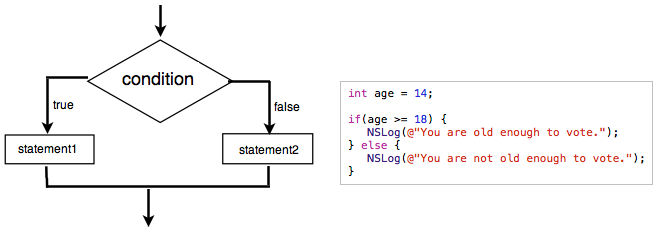The structure theorem states that any program can be written using only three control structures:

1. Sequence
2. Selection
3. Repetition

### The Sequence Statement

The sequence statement refers to doing things in order or sequence, one after the other. Sequence is the natural way in which an iOS application executes instructions. This image shows the flowchart and C code of the sequence statement.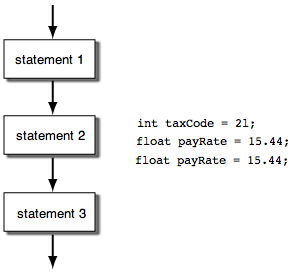### The Selection Control Structure

The C programming language has three forms of the selection control structure you can use in an iOS application.

1. if/else statement
2. else if statement
3. switch statement
##### The if/else Statement

The if/else statement used to execute a statement or block of statements if a condition is true or false. The image below shows a flowchart and C code of the if/else statement. The condition in the flowchart is the expression that is being evaluated. If the condition is true, one or more statements in the if block are executed; otherwise one or more statements in the else block are executed. By the way, the else block of an if/else statement can be omitted if your program logic does not require it.The statement in the else block is executed because the age variable was initialize with the value 14, prior to the if/else statement.

##### The else if Statement

The else if statement is a variation of the if/else statement. You use it when you need to test multiple conditions. The flowchart and C code below shows how. The taxCode was initialize with the value 2 and is evaluated by an the if statement and two else if statements. Since the taxCode is equal to 2; only the statement in the second else if block is executed; which calculate and display salesTax.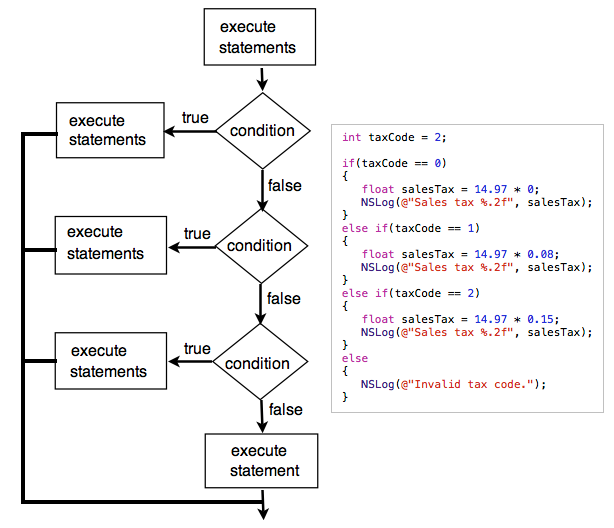##### The switch Statement

The switch statement is used to evaluate a condition, then execute a single statement or block of statements base on the condition result. Here is a flowchart of the switch statement.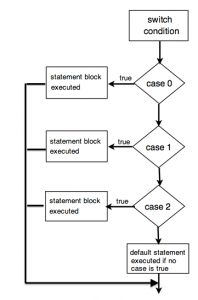It is common programming practice to replace an “else if” statement with a switch statement as demonstrated below.

The if else Statement The Switch Statement
```int taxCode = '22';

if(taxCode == 0)
{
float salesTax = 0;
}
else if(taxCode == 1)
{
float salesTax = 14.97 * "0.08";
}
else if(taxCode == 2)
{
float salesTax = 14.97 * "0.15";
}
else
{
NSLog(@"Invalid tax code.");
}```
```int taxCode = '22';

switch(taxCode){
case 0:
float salesTax = 0;
break;
case 1:
float salesTax = 14.97 * "0.08";
break;
case 2:
float salesTax = 14.97 * "0.15";
break;
default:
NSLog(@"Invalid tax code.");
}```
##### The Conditional (Ternary) Operator

The conditional operator is a simpler alternative to using an if/else conditional statement. We can take the following if/else conditional statement:

```char lightBulbOn = 'n';
if (lightBulbOn == 'y')
NSLog(@"The light bulb is on.");
else
NSLog(@"The light bulb is off.");
```

And replace it with this conditional operator:

```// The parentheses around the conditional statement are not required. I'm using them as a visual guide
outputBox.text = (lightBulbOn == 'y') ? @"The light bulb is on." : @"The light bulb is off.";
```

Syntax
variable = (condition ) ? true : false;

If the value of the lightBulbOn variable equals ‘y’, then the outputBox.text control is set to “The light bulb is on”. Otherwise, it is set to “The light bulb is off.” Once you grasp how the conditional (ternary) operator works it is a great tool to use to trim down your code and simplify your conditional statement.

### The Repetition Control Structure

The C programming language has three forms of the iteration control structure you can use in an iOS application to execute the same code a set number of times.

1. for loop statement
2. while loop statement
3. do loop statement

I will only discuss the first two because the do loop is an inverse of the while loop.

##### The for Loop Statement

The for statement is used to execute the same code a set number of times. The flowchart and C code below shows how.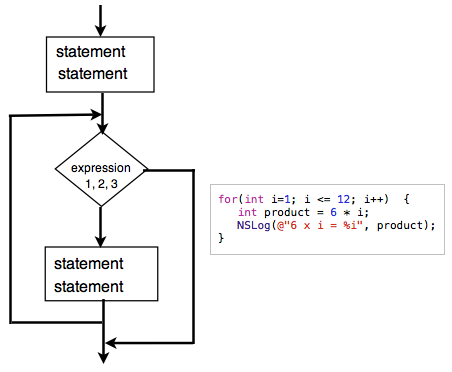First of all, the for statement contain these three expressions:

expression 1 expression 2 expression 3
int i=1; i <=12; i++;

The for statement tells the program to execute expression 1, then evaluate expression 2. If expression 2 is true, then statemens between curly brackets {} are executed. After this, expression 3 is executed, and then expression 2 is evaluated again. If expression 2 is true, then statemens between curly brackets are executed for a second time. The cycle continues until expression 2 is no longer true. Here is output generated by the for statement in above image.

6 x 1 = 6
6 x 2 = 12
6 x 3 = 18
6 x 4 = 24
6 x 5 = 30
6 x 6 = 36
6 x 7 = 42
6 x 8 = 48
6 x 9 = 54
6 x 10 = 60
6 x 11 = 66
6 x 12 = 72

##### The while Loop Statement

The while loop statement evaluates a condition. If it returns true then code in the statement block {} is executed. After the code has executed the condition is evaluated again and the loop will continue until the condition returns false. The statement block must feature code that’ll make the condition false; otherwise, an infinite loop will be created. If the condition returns false when it is first evaluated then the code in the statement block is never executed.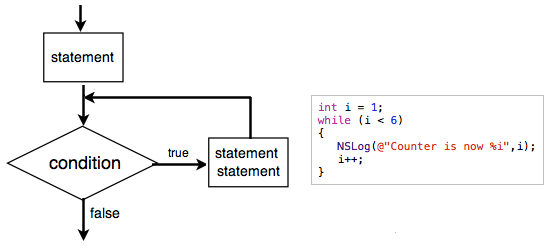C code in above image increment the counter variable value on each iteration of the loop until it reaches five, the evaluation return false, then the loop ends. Here’s what displayed in the Debug window.

counter is now 1
counter is now 2
counter is now 3
counter is now 4
counter is now 5

Leaving out this statement:
i++;
in the statement block will cause an infinite loop. This mean code in the while loop will run for ever.